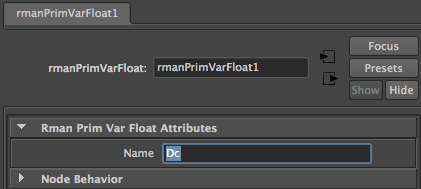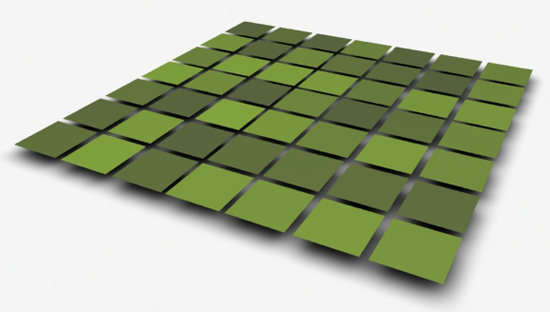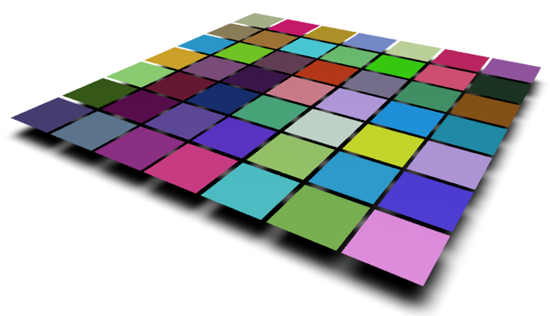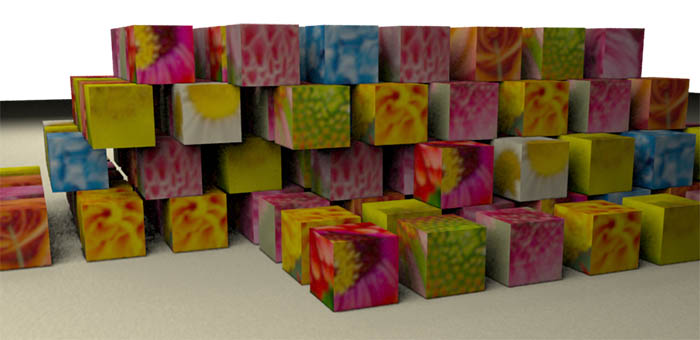##### Topics

Some useful mel procs for adding primvars - primvarProcs.zip

#### Introduction

This tutorial provides three examples of how primvars can be set up and used with RfM and HyperShade. The reader should refer to the tutorial RIB/RSL: Using PrimVars for basic information about primvars.

To use a primvar with a HyperShade material, such as Blinn, requires both the material (aka surface shader) and the geometry to which it is assigned to be set up correctly. The first example shows how a single instance of Blinn and a float primvar can be used to control the diffuse brightness of a grid of polygons. The second example demonstrates how a color primvar can be to used the control the coloration of a grid of polygons. The third example shows how a random selection of texture maps can be applied to a grid of polygons.

#### Example 1 - using a "float" primvar

##### Setting Up the Material

1    In HyperShade create an instance of Blinn and assign it to several surfaces.

2    Scroll to the "Common Material Attributes" tab,
- connect the "Diffuse" parameter to a "Rman Prim Var Float" node.Figure 1

3    Rename the "Rman Prim Var Float Attibute" to "Dc" as shown in figure 2.Figure 2

##### Setting Up the Surfaces

4    Copy and paste the MEL proc, shown in listing 1, into Maya's script window.
Execute the script in order to load it into memory.

Listing 1

 ```global proc addConstFloatPrimVar(string \$shortname, float \$minval, float \$maxval) { string \$sel[] = `ls -sl`; string \$fullname = "rmancF" + \$shortname; for(\$current in \$sel) { string \$shapes[] = `listRelatives -s \$current`; string \$shape = \$shapes; if(attributeExists(\$fullname, \$shape) == 0) { addAttr -ln \$fullname -sn \$fullname -k 1 \$shape; } \$value = rand(\$minval, \$maxval); setAttr (\$shape + "." + \$fullname) \$value; } }```

5    Select the surfaces to which Blinn was assigned,
- execute the following script,
`addConstFloatPrimVar`("Dc", 0.3, 0.8);
The Mel proc will add an attribute named "rmancFDc" to the selected polymesh and will then assign a random value between 0.3 and 0.8. The name of the attribute consists of the prefix "rmancF" and the name given to the primvar in step 6.

When RfM outputs the rib data to the internal or the external version of prman it will "look" for custom attributes beginning with "rmanF". The prefix tells RfM to add a primvar, declared as a "constant float", to each surface. For example,

```PointsGeneralPolygons   [0 1 3 2] "P" [xyz data...]
"constant float Dc" [0.5523]```

Note the prefix acts as a directive to RfM to emit the attribute values as a primvar in the rib stream.

6    Finally, render the scene.Figure 3

#### Naming Conventions

The name assigned in step 3 must match the name of the attribute assigned to geometry in step 5.

#### Example 2 - using a "color" primvar

##### Setting Up the Material

1    In HyperShade create an instance of Blinn and assign it to several surfaces.
2    Scroll to the "Common Material Attributes" tab,
- connect the "Color" parameter to a "Rman Prim Var Color" node.
3    Rename the "Rman Prim Var Color Attibute" to "C".

##### Setting Up the Surfaces

4    Copy and paste the MEL proc, shown in listing 2, into Maya's script window.
Execute the script in order to load it into memory.

Listing 2

 ```global proc addConstColorPrimVar(string \$shortname, vector \$minval, vector \$maxval) { string \$sel[] = `ls -sl`; string \$fullname = "rmanC" + \$shortname; for(\$current in \$sel) { string \$shapes[] = `listRelatives -s \$current`; string \$shape = \$shapes; if(attributeExists(\$fullname, \$shape) == 0) { addAttr -ln \$fullname -sn \$fullname -at "float3" -usedAsColor -k 1 \$shape; addAttr -ln "r" -at "float" -k 1 -parent \$fullname \$shape; addAttr -ln "g" -at "float" -k 1 -parent \$fullname \$shape; addAttr -ln "b" -at "float" -k 1 -parent \$fullname \$shape; } vector \$rgb = rand(\$minval, \$maxval); float \$r = \$rgb.x; float \$g = \$rgb.y; float \$b = \$rgb.z; setAttr (\$shape + "." + \$fullname) -type "float3" \$r \$g \$b; } }```

 5    Select the surfaces to which Blinn was assigned,       - execute the following command,           `addConstColorPrimVar("C", <<0.6, 0.6, 0.6>>, <<1,1,1>>);` The procedure will add an attribute named "rmanCC" to each of the selected surfaces and will then assign a random color between rgb values of 0.6 and 1.0. When RfM outputs the rib data to the internal or the external version of prman it will "look" for custom attributes beginning with "rmanC". The prefix tells RfM to add a primvar, declared as a "constant color", to each surface. For example, ```PointsGeneralPolygons   [0 1 3 2] "P" [xyz data...] "constant color C" [0.520171 0.486881 0.625127]``` 6    Finally, render the scene.Figure 4

#### Example 3 - using a "string" primvar to randomize texture mapping

Unlike the previous examples there is no need to use a primvar utility node because the shader parameter, "XXX_fileTextureName", is automatically "exposed" (ie. inserted into the rib stream) as a result of connecting a `File` node to a material's "Color" or "Surface Color" input. For example, the following snippet,

```shader "RMSGPSurface1_rfm"
"MAP_fileTextureName" "parameter uniform string"
Default value: ""
"diffuseGain" "parameter uniform float"
Default value: 1.000000
"sheen" "parameter uniform float"
Default value: 0.000000
"sheenTint" "parameter uniform float"
Default value: 0.500000```

was generated by the using the utility application "sloinfo" on a compiled instance of RMSGPSurface that had its "Surface Color" connected to a File node named "MAP", figure 5.Figure 5 (File node has been renamed "Map")

This example demonstrates how an arbitrary number of images can be used as texture maps that are randomly assigned to a selection of surfaces. Thus one instance of a material, say, RMSGPSurface can be used to texture many objects with each being randomly assigned a texture.

##### Assumptions

For simplicity the procs shown in listing 3 assume the source images for the texture maps, say, "iris.tif", "rose.tif" etc have been moved to the Maya project's "images" directory. The procs further assume that when the images are converted to textures the .tex files will be stored in the same directory as the source images. The users "images" directory will probably contain image files that are not intended to be used for randomized texture mapping. One of the procs queries a text file ("textureDB.txt") to identify the images that should be used as source maps for the randomization.

##### Setting Up the Material

2     Create a text file named "texturesDB.txt" and save it in the project directory.
3     In "texturesDB.txt" list the images to be used as maps, for example,

```    images/iris.tif
images/rose.tif
images/lily.tif
images/magnolia.tif```

4     Create an instance of a material such as RMSGPSurface or Blinn.
5     Connect a File node to the material's "Color" input.
6     Change the name the file node from "file1" to "MAP".
7     Assign the material to several surfaces.

##### Setting Up the Surfaces

8     Execute the MEL procs shown in listing 3.
9     Ensure the surfaces are "selected" then run the following code,

```string \$paths[] = getTexnames("textureDB.txt");
string \$textures[];
if(size(\$paths) > 0) {
\$textures = makeTextures(\$paths);
}```

The surfaces should render with randomized textures, as shown in figure 6.Figure 6

Listing 3

 ```global proc string[] getTexnames(string \$database) { int \$n = 0; string \$result[]; string \$projPath = `workspace -q -rootDirectory`; string \$dbpath = \$projPath + \$database; int \$fileid = fopen(\$dbpath, "r"); string \$line = fgetline(\$fileid); while(size(\$line) > 0) { \$result[\$n++] = \$projPath + \$line; \$line = fgetline(\$fileid); } fclose(\$fileid); return \$result; } //------------------------------------------------------------ global proc string[] makeTextures(string \$paths[]) { string \$srcpath, \$texpath, \$cmd; string \$result[]; int \$n = 0; for(\$srcpath in \$paths) { \$srcpath = strip(\$srcpath); if(!`filetest -r \$srcpath`) { print("Error: \"" + \$srcpath + "\" does not exist.\n"); continue; } if(!endsWith(\$srcpath, ".tex")) { \$texpath = \$srcpath + ".tex"; \$cmd = "txmake " + \$srcpath + " " + \$texpath; system(\$cmd); if(!`filetest -r \$texpath`) { print("Unable to run \"txmake\" - check your \"PATH\" environment " + "points to RMS/rmantree/bin.\n"); } else print("Info: " + \$cmd + "\n"); \$result[\$n++] = \$texpath; } else { // Texture already exists \$result[\$n++] = \$srcpath; } } return \$result; } //------------------------------------------------------------ global proc addStringPrimVar(string \$shortname, string \$textures[]) { string \$selections[] = `ls -sl`; string \$fullname = "rmanS" + \$shortname; for(\$selection in \$selections) { string \$shapes[] = `listRelatives -s \$selection`; string \$shape = \$shapes; if(attributeExists(\$fullname, \$shape) == 0) { addAttr -ln \$fullname -nn \$fullname -dt "string" \$shape; } // Pick a texture int \$index = rand(0.0, size(\$textures)); string \$name = strip(\$textures[\$index]); setAttr (\$shape + ("." + \$fullname)) -type "string" \$name; print("name >" + \$name + "<"); } }```